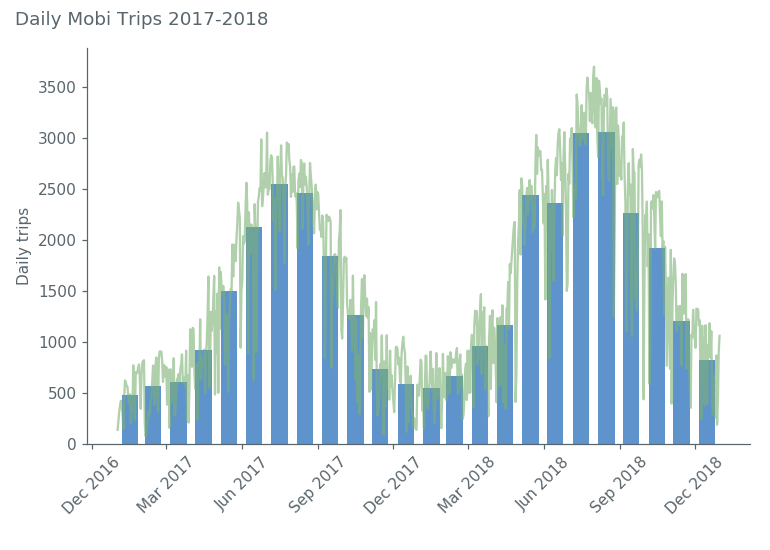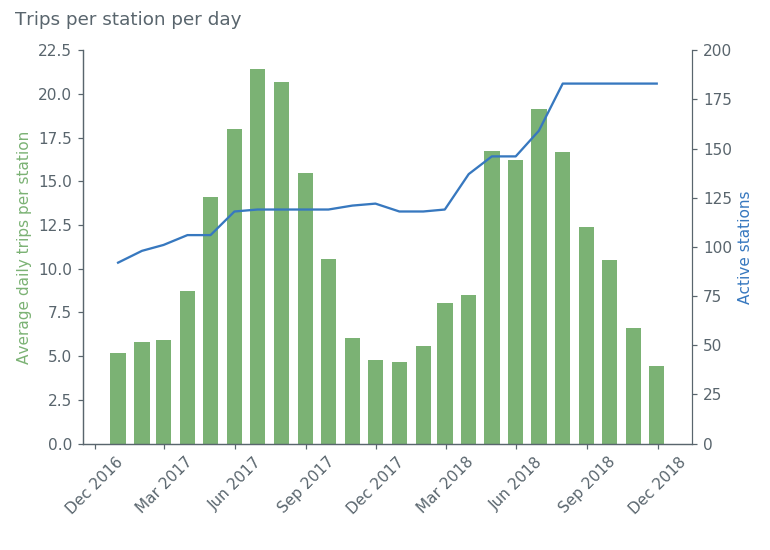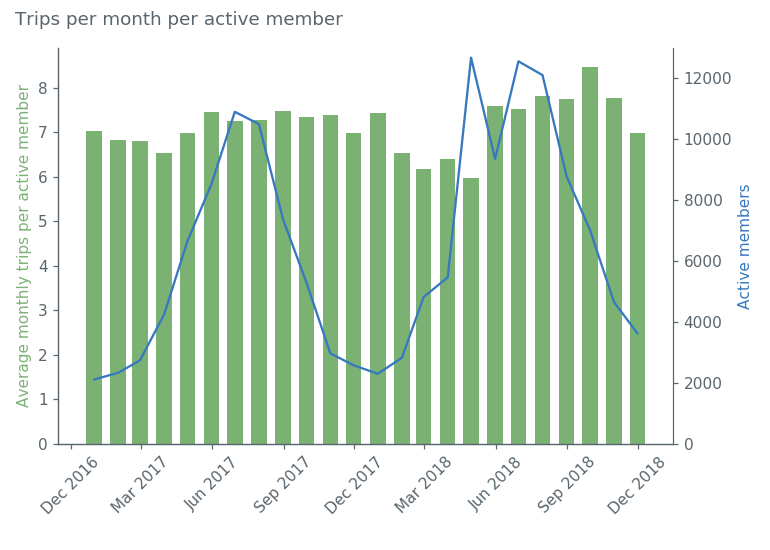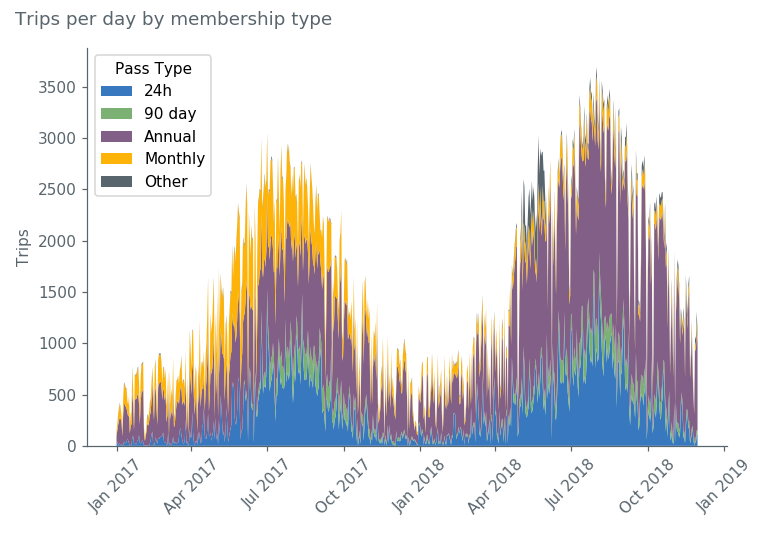# How Vancouver Uses Mobi Bikes

Vancouver's Mobi bikeshare system has been up and running for over 2 years now, and with two full summers of activity it's time to take a look at how exactly Vancouverites are using their bikeshare system.

For over a year, I've been collecting real-time data about Mobi bike trips by monitoring public information about the number of bikes at each station and inferring trip activity based on changes to the number of bikes at each station. This has led to some fun uses: I have live figures updating constantly on my website, a twitter bot tweets out daily stats at @VanBikeShareBot, and a few blog posts.

As handy as those live trip estimates are, they're very much estimates and only give us information about how often certain stations are used. Luckily, Mobi has started publishing open system data. This data set gives us a registry of every Mobi bikeshare trip since the beginning of 2017, current to the end of 2018 as of this writing. With this we have access to trip start and endpoints, trip duration and distance, membership type and more. In this post, I'll summarize some of the things I've learned after spending some time looking into this data.

### A note on the code and data¶

This blog post is written in a Jupyter notebook and contains the code needed to recreate all the figures and data shown. To toggle whether the raw code is displayed, click the blue button below the title of the blog. Beyond the code in this notebook, I rely on a package of python helper functions included in my Mobi repository on github. All the data cleaning done is included in these functions except for standardizing some field names in the raw excel files. To download this post as a notebook, click the "Source" button in the top right corner.

In :
```# Data prep
%matplotlib notebook
import pandas as pd
import matplotlib.pyplot as plt
import sys
sys.path.append('../../mobi/')
import mobi
import numpy as np
from vanweather import get_weather_range
import matplotlib.dates as mdates

df = mobi.prep_sys_df('https://data.mikejarrett.ca/mobi/data/Mobi_System_Data.csv')
df = df.set_index('Departure')
df = df['2017-01':'2018-12']

idx24 = np.array(df['Membership Type']=='24 Hour') | np.array(df['Membership Type']=='Archived Day')
idx90 = np.array(df['Membership Type'].str.contains('90'))
idxvcp = np.array(df['Membership Type'].str.contains('Vancity'))
idxmonthlyall = np.array(df['Membership Type'].str.contains('Monthly'))
idxsingle = np.array(df['Membership Type'].str.contains('Single'))
idxplus = np.array(df['Membership Type'].str.contains('Plus'))
idxvip = np.array(df['Membership Type'] == 'VIP')
idxnotannual = idx24 | idx90 | idxvcp | idxmonthlyall  | idxsingle | idxvip
idx365all = ~idxnotannual
idx365p = idx365all & idxplus
idx365 = idx365all & ~idxplus
```

### Daily trips over time¶

Before diving in, let's take a quick look at total Mobi trips over time. We see the the behaviour we'd expect: substantial seasona variation with some sharp dropoffs that we can assume are particularly rainy days. If you're interested in the factors that influence how many people will ride a Mobi bike on a given day, I've gone into this in more detail in a previous post. Smoothing this out by averaging across each month gives us an idea of the average number of daily trips for each month.

In :
```def tmdf(df):
return df.groupby(pd.Grouper(freq='m')).size().index+pd.DateOffset(-15), df.groupby(pd.Grouper(freq='d')).size().groupby(pd.Grouper(freq='m')).mean()

plot = mobi.plots.Plot()
plot.ax.bar(*tmdf(df),20,alpha=0.8)
plot.ax.set_ylabel("Daily trips")
plot.ax.plot(df.groupby(pd.Grouper(freq='d')).size().index,
df.groupby(pd.Grouper(freq='d')).size(),color=plot.fg_color,alpha=0.6)
plot.ax.xaxis.set_major_formatter(mdates.DateFormatter("%b %Y"))
plot.title("Daily Mobi Trips 2017-2018")
plot.tight_layout()
```In absolute terms, trips clearly up year over year. Which is great! But to get some insight into what might be driving this change let's control for some variable. First, let's normalize by the number of active stations in use in a given month.

In :
```gdf = df.groupby(pd.Grouper(freq='m'))
plot = mobi.plots.Plot()
plot.ax.bar(gdf.size().index-1,
df.groupby(pd.Grouper(freq='d')).size().groupby(pd.Grouper(freq='m')).mean().values/gdf['Departure station'].nunique(),
20,
color=plot.colors
)
plot.ax2 = plot.set_ax_props(plot.ax.twinx())
plot.ax2.plot(df.groupby(pd.Grouper(freq='m'))['Departure station'].nunique().index-1,
df.groupby(pd.Grouper(freq='m'))['Departure station'].nunique().values)
plot.ax.spines['right'].set_visible(True)
plot.ax2.set_ylabel("Active stations")
plot.ax2.set_ylim((0,200))
plot.ax.xaxis.set_major_formatter(mdates.DateFormatter("%b %Y"))
plot.ax.set_ylabel("Average daily trips per station")
plot.ax.yaxis.label.set_color(plot.fg_color)
plot.ax2.yaxis.label.set_color(plot.fg_color2)
plot.title("Trips per station per day")
plot.tight_layout()
```I don't think it's a bad thing that the trips per station metric is down year over year -- spreading outside the core of Vancouver necessarily means less use at new stations, and there's lots of long-term value in having lots of stations that people can use when needed even if they're not in the highest demand areas.

Similarly, we might wonder how much of trip growth is driven by new members versus members taking more frequent trips. I don't have registration information for Mobi users so I don't know who's account is active at any given time, so I'll consider a member "active" if they've taken at least one trip in a given month. We can then normalize monthly trips by active members to look at how many trips the average member takes per month.

In :
```gdf = df.groupby(pd.Grouper(freq='m'))
plot = mobi.plots.Plot()
plot.ax.bar(gdf.size().index-1,
gdf.size()/gdf['Account'].nunique(),
20,
color=plot.colors
)
plot.ax2 = plot.set_ax_props(plot.ax.twinx())
plot.ax2.plot(df.groupby(pd.Grouper(freq='m'))['Account'].nunique().index-1,
df.groupby(pd.Grouper(freq='m'))['Account'].nunique().values)
plot.ax.spines['right'].set_visible(True)
plot.ax2.set_ylabel("Active members")
plot.ax2.set_ylim((0,13000))
plot.ax.xaxis.set_major_formatter(mdates.DateFormatter("%b %Y"))
plot.ax.set_ylabel("Average monthly trips per active member")
plot.ax.yaxis.label.set_color(plot.fg_color)
plot.ax2.yaxis.label.set_color(plot.fg_color2)
plot.title("Trips per month per active member")
plot.tight_layout()
```Monthly trips per active member has stayed reasonably constant over time, maybe with a slight uptick in recent months. This tells me that users joining Mobi are behaving fairly consistently over time, and the uptick in trips is due to membership growth.

### Different members types take different trips¶

I want to focus on the new information we have available with the official system data, so instead of just looking at raw trip counts let's instead look at how trips are distrubted over time by membership type. According to the system data, there's over a dozen membership types that have been used at one point or another, but they broadly break down into a few groups: Daily pass, monthly pass, 90 day or annual pass. All these entitle the holder to unlimited 30 minute trips for the duration of the pass. Monthly, 90 day and annual members also have the option of buying a "plus" account, which entitles them to 60 minute trips. There are a handful of other membership types -- VanCity community pass, VIP, etc -- which I'll lump together as "other".

In :
```def tddf(df):
tddf = df.groupby(pd.Grouper(freq='d')).size()
tddf = tddf.reindex(pd.date_range('01-01-2017', '11-30-2018'),fill_value=0)
return tddf

plot = mobi.plots.Plot()
sdf = pd.DataFrame(index=tddf(df).index.values,
data={'Annual':tddf(df[idx365all]).values,
'24h':tddf(df[idx24]).values,
'Monthly':tddf(df[idxmonthlyall]).values,
'90 day':tddf(df[idx90]),
'Other':tddf(df[~idx24 & ~idx365all & ~idx90 & ~idxmonthlyall])})
plot.ax.stackplot(sdf.index.values,sdf.T,labels=sdf.columns,colors=plot.colors)
plot.ax.legend(loc=2,title="Pass Type")
plot.ax.xaxis.set_major_formatter(mdates.DateFormatter("%b %Y"))
plot.title("Trips per day by membership type")
plot.tight_layout()
```A couple main takeaways here. First, we see that the main membership types offered by Mobi have changed over type. Monthly memberships were phased out in favour of 90 day passes, and single trip passes were dropped.

The growth in trips in 2018 over 2017 looks to be driven by annual members more than single day pass users. Annual members are also the driving force in keeping the system active during the cold and rainy months between October and April.

Let's zoom in on a shorter timeframe to see what behaviour we see on a day to day basis.

In :
```def thdf(df):
return df.groupby(pd.Grouper(freq='H')).size()
idx20180811 = np.array(df.index > '2018-08-11') & np.array(df.index < '2018-08-18')
plot = mobi.plots.Plot(5)

idxs = [idx20180811 & idx365all,idx20180811 & idx90,idx20180811 & idxmonthlyall,idx20180811 & idx24,idx20180811 & ~idx365all & ~idx90 & ~idxmonthlyall & ~idx24]
labels = ['Annual members','90 day members','Monthly members','24h pass','Other']
for idx,label,ax,c in zip(idxs,labels,plot.ax,plot.colors):
p, = ax.plot(thdf(df[idx]),label=label,color=c)
#ax.fill_betweenx(list(df[idx].index),list(df[idx]))
ax.legend(loc=2)
ax.xaxis.set_major_formatter(mdates.DateFormatter(""))
ax.set_ylabel('Trips/hour')
ax.xaxis.set_major_formatter(mdates.DateFormatter("%A"))

plot.f.set_figheight(10)
plot.title("Hourly trips by membership type")
plot.tight_layout()

## Stacked line chart (cool but too confusing I think)
# def thdf(df):
#     return df.groupby(pd.Grouper(freq='H')).size()
# idx20180811 = np.array(df.index > '2018-08-11') & np.array(df.index < '2018-08-18')
# plot = mobi.plots.Plot()
# sdf = pd.DataFrame(index=thdf(df[idx20180811]).index.values,data={'Annual':thdf(df[idx20180811 & idx365all]).values,'24h':thdf(df[idx20180811 & idx24]).values,'Monthly':thdf(df[idx20180811 & idxmonthlyall]),'90 day':thdf(df[idx20180811 & idx90]),'Other':thdf(df[~idx24 & ~idx365all & ~idx90 & idx20180811])},)
# plot.ax.stackplot(sdf.index.values,sdf.T,labels=sdf.columns,colors=plot.colors)
# plot.ax.legend(loc=2,title="Pass Type")
# plot.ax.xaxis.set_major_formatter(mdates.DateFormatter("%A"))
# plot.ax.set_ylabel('Trips/hour')
# plot.f.tight_layout()
```